# uitable

Create table user interface component

## Syntax

``uit = uitable``
``uit = uitable(parent)``
``uit = uitable(___,Name,Value)``

## Description

````uit = uitable` creates a table UI component in the current figure and returns the `Table` UI component object. If there is no figure available, MATLAB® calls the `figure` function to create one.```
````uit = uitable(parent)` creates a table in the specified parent container. The parent container can be a figure created with either the `figure` or `uifigure` function or a child container such as a panel.```

example

````uit = uitable(___,Name,Value)` specifies table properties using one or more name-value arguments. Use this option with any of the input argument combinations in the previous syntaxes. Property values for a table UI component vary slightly depending on whether the app is created with the `figure` or `uifigure` function.```

## Examples

collapse all

Create a table UI component that displays a 10-by-3 array of random integers.

```fig = uifigure; uit = uitable(fig,"Data",randi(100,10,3));```Create a table array `t` with different data types by reading data from a file. Select the first 15 rows of four variables from `t`.

```t = readtable("patients.xls"); vars = ["Age","Systolic","Diastolic","Smoker"]; t = t(1:15,vars);```

Create a table UI component to display the tabular data. The data type determines how the data appears in the component. For example, logical data displays as a check box. For more information, see Display Tabular Data in Apps.

```fig = uifigure; uit = uitable(fig,"Data",t,"Position",[20 20 350 300]);```Display and programmatically update data in a table UI component.

Create a table array by reading in tsunami data from a file, and display a subset of the data in a table UI component.

```t = readtable("tsunamis.xlsx"); vars = ["Year","MaxHeight","Validity"]; t = t(1:20,vars); fig = uifigure; uit = uitable(fig,"Data",t);```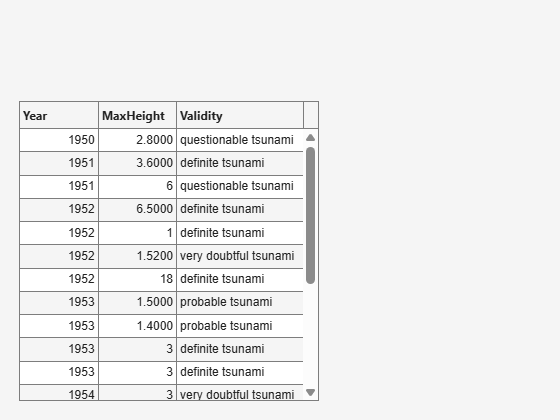Update the validity of the tsunami in the first row by editing the `Data` property of the table UI component.

`uit.Data.Validity(1) = {'definite tsunami'};`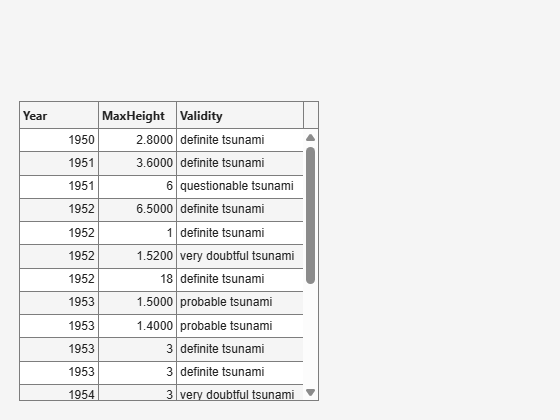Convert the maximum height data from meters to feet by accessing and modifying the data in the `MaxHeight` variable.

`uit.Data.MaxHeight = uit.Data.MaxHeight*3.281;`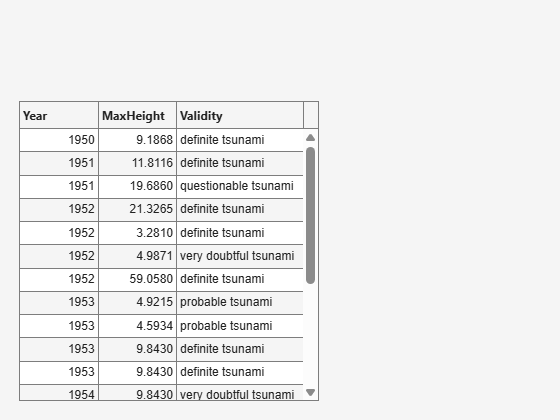Create an app that allows users to sort and edit table data, and that updates a data visualization when data is changed.

In a file named `tableDataApp.m`, write a function that implements the app:

• Create a `table` array of tsunami data.

• Create a UI figure with a grid layout manager.

• Create a sortable and editable table UI component in the figure. Store the `table` array in the component `Data` property.

• Create a bubble chart to visualize the tsunami data, where the coordinates of a bubble represent the latitude and longitude of the tsunami and the size of the bubble represents the maximum height.

• Update the bubble chart when the app user sorts columns or edits cells in the table UI component by writing a `DisplayDataChangedFcn` callback. For more information about callbacks, see Create Callbacks for Apps Created Programmatically.

```function tableDataApp % Create table array t = readtable("tsunamis.xlsx"); vars = ["Latitude","Longitude","MaxHeight"]; t = t(1:20,vars); % Create UI figure fig = uifigure; fig.Position(3:4) = [722 360]; gl = uigridlayout(fig,[1 2]); % Create table UI component uit = uitable(gl); uit.Data = t; uit.ColumnSortable = true; uit.ColumnEditable = [false false true]; % Create bubble chart ax = geoaxes(gl); lat = t.Latitude; long = t.Longitude; sz = t.MaxHeight; bubblechart(ax,lat,long,sz) % Specify table callback uit.DisplayDataChangedFcn = @(src,event) updatePlot(src,ax); end function updatePlot(src,ax) t = src.DisplayData; lat = t.Latitude; long = t.Longitude; sz = t.MaxHeight; bubblechart(ax,lat,long,sz) end ```

Run the app.

`tableDataApp`A sortable column displays arrows in the header when you point to it. Sort the table by the maximum height of the tsunamis.Edit the maximum height of the tsunami in the second row to be 30 meters by double-clicking the table cell and entering the new height. The bubble chart updates in response.Since R2019b

Style rows, columns, or cells of a table UI component using the `uistyle` and `addStyle` functions. Styles are only supported when the table UI component is in a figure created with the `uifigure` function.

Read tsunami sample data into the workspace as a table array. Then, create a table UI component to display the data.

```tdata = readtable("tsunamis.xlsx"); vars = ["Year","Month","Day","Hour", ... "Cause","EarthquakeMagnitude"]; tdata = tdata(1:20,vars); fig = uifigure("Position",[500 500 760 360]); uit = uitable(fig, ... "Data",tdata, ... "Position",[20 20 720 320]); ```

Use the `ismissing` function to get a logical array of the table elements that contain missing values. Find the row and column subscripts for the elements that have `NaN` values. Finally, create a red background color style and add it to the cells in the table with `NaN`.

```styleIndices = ismissing(tdata); [row,col] = find(styleIndices); s = uistyle("BackgroundColor",[1 0.6 0.6]); addStyle(uit,s,"cell",[row,col]);```Create an app that plots data on a map when the app user selects the data in a table.

In a file named `selectTsunamis.m`, write a function that implements the app:

• Create a UI figure with a table UI component and a geographic bubble chart in a grid layout manager.

• Configure the table UI component. Store the tsunami data in the `Data` property, and let users select multiple rows by setting the `SelectionType` and `MultiSelect` properties.

• Specify a `SelectionChangedFcn` callback that updates the bubble chart when the app user changes the table selection. The `plotTsunami` callback function plots a bubble for each selected row, where the size of the bubble represents the maximum tsunami height. For more information about callbacks, see Create Callbacks for Apps Created Programmatically.

```function selectTsunamis % Load data t = readtable("tsunamis.xlsx"); vars = ["Latitude","Longitude","MaxHeight"]; t = t(1:20,vars); % Create UI components fig = uifigure("Position",[100 100 800 350]); gl = uigridlayout(fig,[1 2]); gl.ColumnWidth = {'1x','2x'}; tbl = uitable(gl); gb = geobubble(gl,[],[]); % Configure table tbl.Data = t; tbl.SelectionType = "row"; tbl.Multiselect = "on"; tbl.SelectionChangedFcn = @(src,event) plotTsunami(src,event,gb); end % Plot tsunami data for each selected row function plotTsunami(src,event,gb) rows = event.Selection; data = src.Data(rows,:); gb.LatitudeData = data.Latitude; gb.LongitudeData = data.Longitude; gb.SizeData = data.MaxHeight; end ```

Run the app.

`selectTsunamis`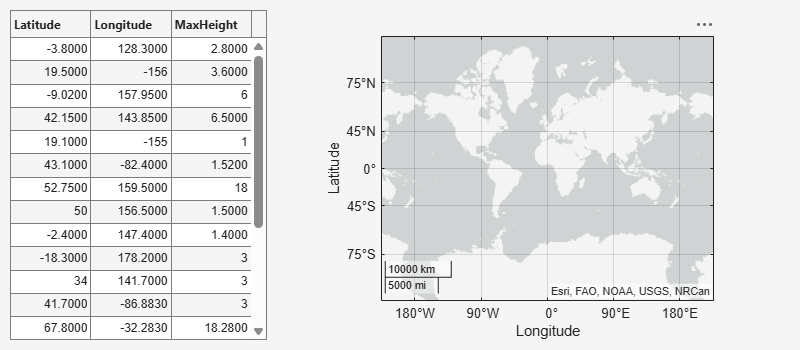Select multiple table rows by holding Ctrl while clicking. The plot updates with the selected tsunami data.Since R2021a

Programmatically scroll to a row, column, or cell of a table UI component using the `scroll` function. Programmatic scrolling is only supported when the table UI component is in a figure created with the `uifigure` function.

Read sample patient data into the workspace as a table array. Then, create a table UI component to display the data.

```tdata = readtable("patients.xls"); vars = ["Age","Systolic","Diastolic","Smoker"]; tdata = tdata(1:40,vars); fig = uifigure; uit = uitable(fig,"Data",tdata); uit.RowName = "numbered";```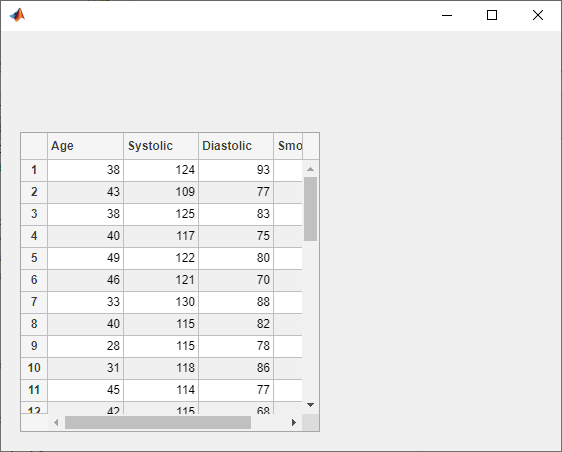Scroll to the 25th row of the table.

`scroll(uit,"row",25)`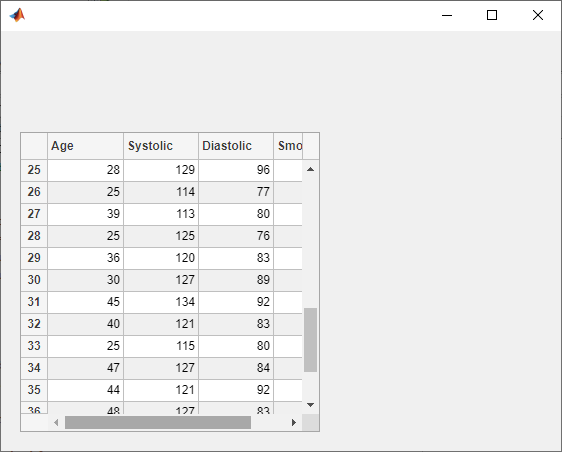## Input Arguments

collapse all

Parent container, specified as a figure created with either the `figure` or `uifigure` function, or a child container in a figure, such as a panel, tab, button group, or grid layout manager.

### Name-Value Arguments

Specify optional pairs of arguments as `Name1=Value1,...,NameN=ValueN`, where `Name` is the argument name and `Value` is the corresponding value. Name-value arguments must appear after other arguments, but the order of the pairs does not matter.

Example: `uitable(Data=[1 2 3; 4 5 6])` creates a table UI component with the specified data.

Before R2021a, use commas to separate each name and value, and enclose `Name` in quotes.

Example: `uitable("Data",[1 2 3; 4 5 6])` creates a table UI component with the specified data.

Note

The properties listed here are a subset of the available properties. For the full list, see Table Properties.

Table data, specified as one of the following types of array:

• Table array (`uifigure`-based apps only) — Displays any combination of data types that `table` arrays support, such as `datetime`, `duration`, and `categorical`.

• Numeric array — Displays numeric values such as `double` or `single`.

• Logical array — Displays check boxes. `true` values correspond to selected boxes, whereas `false` values display cleared boxes.

• Cell array — Displays any combination of numeric, logical, or character array values.

• String array — Displays characters and text.

• Cell array of character vectors — Displays characters and text.

To prevent warnings or `NaN` values that display when users enter invalid data into an editable cell, write a `CellEditCallback` function to convert the data to the appropriate type. When a user edits a cell, the `Data` property updates.

#### Specify a Table Array

In App Designer and apps created using the `uifigure` function, you can specify the `Data` property as a table array. Table arrays provide a convenient way to store tabular data as a MATLAB variable. The `table`, `readtable`, and `array2table` functions create table arrays. By contrast, the `uitable` function creates a `Table` UI component (a user interface component for an app).

When you specify the `Data` property of a `Table` UI component as a table array, then MATLAB sets the format of the `Table` UI component automatically based on the values in the table array:

• By default, the column names displayed in the app match the `VariableNames` property of the table array. Changing the `ColumnName` property of the `Table` UI component updates the UI, but it does not update the variable names in the table array.

• By default, the row names displayed in the app match the `RowName` property of the table array. Changing the `RowName` property of the `Table` UI component updates the UI, but it does not update the row names in the table array.

• The data type of each table array variable controls formatting for the corresponding column in the app. If you try to set the `ColumnFormat` property, MATLAB returns a warning.

For more information on displaying table array data, see Display Tabular Data in Apps.

#### Specify Numeric, Logical, Cell, String Array, or Cell Array of Character Vectors

Use the `ColumnFormat` property to specify the format for data that is a numeric, logical, cell, or string array, or a cell array of character vectors. If data is edited and results in a mismatch between the data type of the data and the `ColumnFormat` property, MATLAB converts the data or displays a warning. See the `ColumnFormat` property description for more information.

Width of table columns, specified as `'auto'` or as a `1`-by-`n` cell array of character vectors, strings, and numeric values. In `uifigure`-based apps, you can additionally specify the column width as `'1x'` or `'fit'`.

• Automatic widths — Specify `'auto'` to have MATLAB calculate the widths of the columns automatically using several factors, one of which is the `ColumnName` property value.

• Fit widths to content (`uifigure`-based apps only) — Specify a value of `'fit'` to configure columns to strictly adjust widths to fit column names and data. This setting allows narrower columns than `'auto'` does.

• Uniform widths (`uifigure`-based apps only) — Specify a value of `'1x'` to make all columns the same width, dividing the available space equally.

• Fixed widths — Specify a cell array of numeric values that define the column widths in pixel units.

• Combinations — You can combine fixed and variable column widths in a cell array. Each element in the cell array corresponds to a column in the table. If the cell array you specify has fewer values than the number of columns, then the columns with no specified value keep the default value of `'auto'`. If the array has more values than the number of columns, MATLAB ignores the extra values.

• Weighted variable widths (`uifigure`-based apps only) — Specify a cell array with character vectors or strings composed of a number concatenated with an `'x'` (for example, `'2x'`, `'3x'`, etc.). The x-factor of each column sets that column width proportionally with respect to the others, with consideration for the remaining space in the UI table.

If a user interactively resizes a table column in a running app, the resized column width persists even if you later update the `ColumnWidth` property.

Example: `uit = uitable(uifigure,'ColumnWidth','auto','Data',[1 2 3;4 5 6])`

Example: `uit = uitable(uifigure,'ColumnWidth','fit','Data',[1 2 3;4 5 6])`

Example: `uit = uitable(uifigure,'ColumnWidth',{64,60,40},'Data',[1 2 3;4 5 6])`

Example: `uit = uitable(uifigure,'ColumnWidth',{'2x','1x','1x'},'Data',[1 2 3;4 5 6])`

Example: `uit = uitable(uifigure,'ColumnWidth',{64,"auto",40},'Data',[1 2 3;4 5 6])`

Example: `uit = uitable(uifigure,'ColumnWidth',{'fit','1x','3x'},'Data',[1 2 3;4 5 6])`

Ability to edit column cells, specified as:

• An empty logical array (`[]`) — No columns are editable.

• A logical 1-by-`n` array — This array specifies which columns are editable. The value of `n` is equal to the number of columns in the table. Each value in the array corresponds to a table column. A value of `true` in the array makes the cells in that column editable. A value of `false` makes the cells in that column uneditable. If the array has more values than the number of columns, MATLAB ignores the excess values. If the array has fewer values than the number of columns, then the columns with no specified value are not editable.

• A logical scalar — The entire table is editable or uneditable.

When a user edits a cell, the `Data` property updates.

Example: `uit = uitable(uifigure,'Data',rand(10,3),'ColumnEditable',[false true true])`

Example: `uit = uitable(uifigure,'Data',rand(10,3),'ColumnEditable',false)`

To enable users to interact with the controls in table columns that contain check boxes or pop-up menus, set the `ColumnEditable` property to `true`.

If the `Data` property is a table array, then any variables that are multicolumn or contain non-editable data types, like `duration`, are not editable in the running app even when the `ColumnEditable` property is `true`. Table array variables that contain mixed data types in a cell array are editable in the running app, as long as the data types are editable.

Cell edit callback function, specified as one of these values:

• A function handle.

• A cell array in which the first element is a function handle. Subsequent elements in the cell array are the arguments to pass to the callback function.

• A character vector containing a valid MATLAB expression (not recommended). MATLAB evaluates this expression in the base workspace.

Use this callback function to perform calculations or validate input when the app user changes the contents of a table cell.

This callback function can access specific information about the user’s interaction with the cell (such as the cell indices). MATLAB passes this information in a `CellEditData` object as the second argument to your callback function. In App Designer, the argument is called `event`. You can query the object properties using dot notation. For example, `event.Indices` returns the indices of the selected cell. The `CellEditData` object is not available to callback functions specified as character vectors.

The following table describes properties of the `CellEditData` object.

Property

Description

`Indices`

This is a 1-by-2 array containing the row and column indices of the cell the user edited in the running app. When a column is sorted, `Indices` returns the original 1-by-2 array of a cell before it was sorted—`DisplayIndices` returns the new location of the edited cell that displays visually in the sorted table.

`DisplayIndices`

This is a 1-by-2 array containing the row and column indices corresponding to the location of the edited cell in the display of the sorted table. If a user does not sort columns, then `DisplayIndices` has the same content as the `Indices` property.

`PreviousData`

This is the previous cell data. The default is an empty matrix, `[]`.

`EditData`

This is the user-entered value.

`NewData`

This is the value that MATLAB wrote to the `Data` property array.

The `NewData` property is empty if MATLAB detects an error in the user-entered data.

`Error`

This is the error message returned if MATLAB detects an error in the user-entered data.

The `Error` property is empty when MATLAB successfully writes the value to the `Data` property.

If the `Error` property is not empty, then the `CellEditCallback` can display the message, or it can attempt to fix the problem.

`Source`

Component executing the callback.

`EventName`

`'CellEdit'`.

When the user edits a table cell, MATLAB performs these steps:

1. Tries to store the new value into the `Data` property of the table

2. Calls the `CellEditCallback` function (if it exists)

If the value results in an error and there is no `CellEditCallback` function, then the cell data reverts to its previous value and no error displays.

Location and size of the table, specified as a four-element vector of the form `[left bottom width height]`. This table describes each element in the vector.

ElementDescription
`left`Distance from the inner left edge of the parent container to the outer left edge of the table
`bottom`Distance from the inner bottom edge of the parent container to the outer bottom edge of the table
`width`Distance between the right and left outer edges of the table
`height`Distance between the top and bottom outer edges of the table

All measurements are in units specified by the `Units` property.

The `Position` values are relative to the drawable area of the parent container. The drawable area is the area inside the borders of the container and does not include the area occupied by decorations such as a menu bar or title.

Units of measurement, specified as one of the values in this table.

Units ValueDescription
`'pixels'` (default)

Distances in pixels are independent of your system resolution on Windows® and Macintosh systems:

• On Windows systems, a pixel is 1/96th of an inch.

• On Macintosh systems, a pixel is 1/72nd of an inch.

On Linux® systems, the size of a pixel is determined by your system resolution.

`'normalized'`

These units are normalized with respect to the parent container. The lower-left corner of the container maps to `(0,0)` and the upper-right corner maps to `(1,1)`.

`'inches'`Inches.
`'centimeters'`Centimeters.
`'points'`Points. One point equals 1/72nd of an inch.
`'characters'`

These units are based on the default uicontrol font of the graphics root object:

• Character width = width of the letter `x`.

• Character height = distance between the baselines of two lines of text.

To access the default uicontrol font, use `get(groot,'defaultuicontrolFontName')` or `set(groot,'defaultuicontrolFontName')`.

The recommended value is `'pixels'`, because most MATLAB app building functionality measures distances in pixels. You can create a table that rescales based on the size of the parent container by parenting the table to a grid layout manager created using the `uigridlayout` function. For more information, see Lay Out Apps Programmatically.

## Version History

Introduced in R2008a

expand all